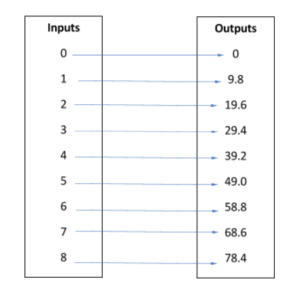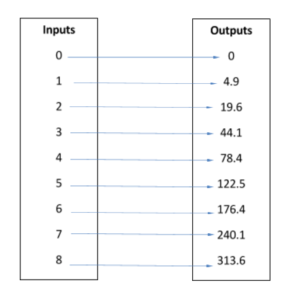## Putting It Together: Function Basics

At the beginning of the module, you were considering Galileo’s famous experiment in which he dropped, or thought about dropping, two balls of different masses from the top of the Tower of Pisa. And you looked at data describing a falling object. Now that you learned about functions, let’s take another look at the data.

 Time (s) 0 1 2 3 4 5 6 7 8 Velocity (m/s) 0 9.8 19.6 29.4 39.2 49 58.8 68.6 78.4 Distance (m) 0 4.9 19.6 44.1 78.4 122.5 176.4 240.1 313.6

Chart shows the correlation between time (seconds 1-8), velocity and distance.

Consider the set of ordered pairs relating time to velocity.

{(0, 0), (1, 9.8), (2, 19.6), (3, 29.4), (4, 39.2), (5, 49.0), (6, 58.8), (7, 68.6), and (8, 78.4)}

Is the relation a function? Indeed it is. Each input value corresponds to only one output value.Now consider the set of ordered pairs relating time to distance.

{(0, 0), (1, 4.9), (2, 19.6), (3, 44.1), (4, 78.4), (5, 122.5), (6, 176.4), (7, 240.1), and (8, 313.6)}

As before, this relation is also a function.Now that you know that both velocity and distance can be described by functions, you can evaluate them from the table.

• Suppose velocity as a function of time is represented as $V(t)$.  Evaluate and explain $V(4)$. To evaluate, find the value of the function at 4 seconds.  At 4 seconds, velocity is 39.2 m/s.  Therefore, $V(4)=39.2$.
• Similarly, solve for $t$ when $V(t)=49.0$.  Find 49.0 m/s on the table and read the related time, 5 s. That means that the velocity is 49.0 m/s after 5 seconds so $V(5)=49.0$.
• Suppose now that the distance is represented as $D(t)$.  Evaluate and explain $D(6)$. To evaluate, find the value of the function at 6 seconds. It is 176.4 m, which means that the falling object travels 176.4 m in 6 seconds.
• We can also solve $D(t)=240.1$.  Find 240.1 m in the table and read the related time, which is 7 seconds.  So $D(7)=240.1$.

You can also use the table to try to determine the function formulas. Begin by reading across the velocity data to look for a pattern. Each value is 9.8 times the number of seconds.  So the velocity as a function of time can be represented by the formula $V(t)=at$, where a is acceleration (in this case due to gravity) and $t$ is time.

Finding the function formula for distance is a little trickier. The distance as a function of time can be represented by the formula , where $a$ is acceleration and $t$ is time.

Now that you know the function formulas, you can evaluate them for any value of time.  Suppose it takes an object dropped from a higher floor of the Burj Khalifa 10 seconds to fall to the ground. How fast would the object be traveling when it hit, and how far would it have fallen in that time?

We can evaluate the functions for velocity and distance at 10 seconds to answer these questions.

Substitute 10 seconds for $t$ in each function. Remember that acceleration due to gravity is 9.8 m/s2.

 $V(t)=at$ $D(10)={\Large\frac{1}{2}}at^2$ $V(10)=a(10)$ $D(10)={\Large\frac{1}{2}}a(10)^2$ $V(10)=(9.8)(10)$ $D(10)={\Large\frac{1}{2}}(9.8)(10)^2$ $V(10)=98$ $D(10)=490$

Add these values to the table to the table and what do you see? Velocity and distance really start to increase the longer the object falls. Let’s evaluate the functions at 13 seconds and add those results to the table as well.

 Time (s) 0 1 2 3 4 5 6 7 8 10 13 Velocity (m/s) 0 9.8 19.6 29.4 39.2 49 58.8 68.6 78.4 98 127.4 Distance (m) 0 4.9 19.6 44.1 78.4 122.5 176.4 240.1 313.6 490 828.1

Chart shows the correlation between time (seconds 1-8, 10 and 13), velocity and distance.

That means that it would take about 13 seconds for an object to fall the tremendous height of the tallest building in the world, and it would be traveling about 127 m/s. That is almost 300 miles per hour! You certainly don’t want to be under that when it falls. Thankfully, due to your knowledge of functions, you would know to stay far away.